# Linear Spaces¶

## Definition and basic properties¶

A linear space over a field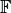is a set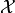, endorsed with the operations of vector addition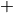” and scalar multiplication” which are required to fullfill certain properties, usually called axioms. To emphasize the importance of all ingredients, vector spaces are often written as tuples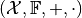. We always assume that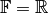or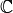.

In the following, we list the axioms, which are required to hold for arbitrary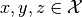and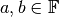.

 Associativity of addition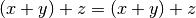Commutativity of addition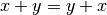Existence of a neutral element of addition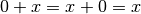Existence of inverse elements of addition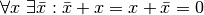Compatibility of multiplications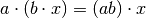Neutral scalar is the neutral element of scalar multiplication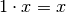Distributivity with respect to vector addition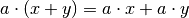Distributivity with respect to scalar addition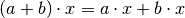Of course, the inverse element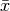is usually denoted with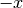.

## Metric spaces¶

The vector spaceis called a metric space if it is additionally endorsed with a distance function or metric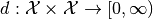with the following properties for all: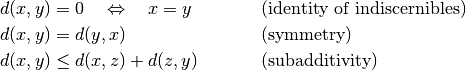We call the tuple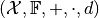a Metric space.

## Normed spaces¶

A function onintended to measure lengths of vectors is called a norm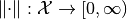if it fulfills the following conditions for all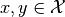and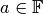: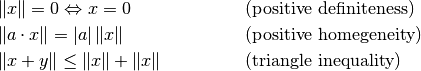A tuple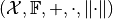fulfilling these conditions is called Normed vector space. Note that a norm induces a natural metric via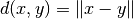.

## Inner product spaces¶

Measure angles and defining notions like orthogonality requires the existence of an inner product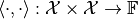with the following properties for alland: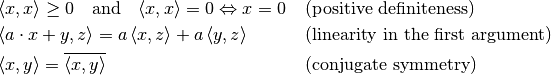The tuple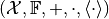is then called an Inner product space. Note that the inner product induces the norm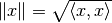.

## Cartesian spaces¶

We refer to the space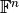as the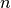-dimensional Cartesian space over the field. We choose this notion since Euclidean spaces are usually associated with the Euclidean norm and distance, which are just (important) special cases. Vector addition and scalar multiplication inare, of course, realized with entry-wise addition and scalar multiplication.

The natural inner product inis defined as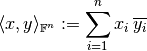and reduces to the well-known dot product if. For the norm, the most common choices are from the family of p-norms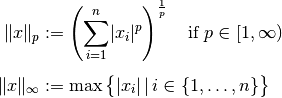with the standard Euclidan norm for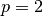. As metric, one usually takes the norm-induced distance function, although other choices are possible.

## Weighted Cartesian spaces¶

In the standard definition of inner products, norms and distances, all components of a vector are have the same weight. This can be changed by using weighted versions of those functions as described in the following.

Let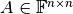be a Hermitian square and positive definite matrix, in short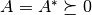. Then, a weighted inner product is defined byWeighted norms can be defined in different ways. For a general norm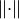, a weighted version is given by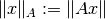For the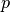-norms with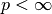, the definition is usually changed to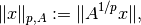where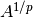is the-th root of the matrix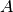. The reason for this definition is that for, this version is consistent with the inner product since.

### Remark on matrices as operators¶

A matrix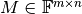can be regarded as a linear operator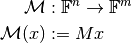It is well known that in the standard case of a Euclidean space, the adjoint operator is simply defined with the conjugate transposed matrix: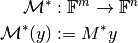However if the spacesand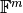have weighted inner products, this identification is no longer valid. If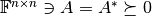and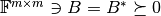are the weighting matrices of the inner products, we get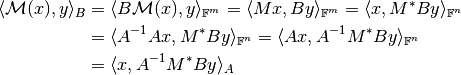Thus, the adjoint of the matrix operator between the weighted spaces is rather given as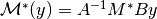.